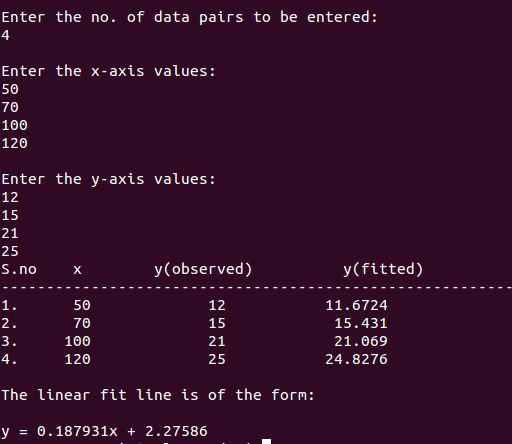# C++ Program to Linear Fit the data using Least Squares Method

```//Linear Fit
#include<iostream>
#include<iomanip>
#include<cmath>
using namespace std;
int main()
{
int i,j,k,n;
cout<<"\nEnter the no. of data pairs to be entered:\n";        //To find the size of arrays
cin>>n;
double x[n],y[n],a,b;
cout<<"\nEnter the x-axis values:\n";                //Input x-values
for (i=0;i<n;i++)
cin>>x[i];
cout<<"\nEnter the y-axis values:\n";                //Input y-values
for (i=0;i<n;i++)
cin>>y[i];
double xsum=0,x2sum=0,ysum=0,xysum=0;                //variables for sums/sigma of xi,yi,xi^2,xiyi etc
for (i=0;i<n;i++)
{
xsum=xsum+x[i];                        //calculate sigma(xi)
ysum=ysum+y[i];                        //calculate sigma(yi)
x2sum=x2sum+pow(x[i],2);                //calculate sigma(x^2i)
xysum=xysum+x[i]*y[i];                    //calculate sigma(xi*yi)
}
a=(n*xysum-xsum*ysum)/(n*x2sum-xsum*xsum);            //calculate slope
b=(x2sum*ysum-xsum*xysum)/(x2sum*n-xsum*xsum);            //calculate intercept
double y_fit[n];                        //an array to store the new fitted values of y
for (i=0;i<n;i++)
y_fit[i]=a*x[i]+b;                    //to calculate y(fitted) at given x points
cout<<"S.no"<<setw(5)<<"x"<<setw(19)<<"y(observed)"<<setw(19)<<"y(fitted)"<<endl;
cout<<"-----------------------------------------------------------------\n";
for (i=0;i<n;i++)
cout<<i+1<<"."<<setw(8)<<x[i]<<setw(15)<<y[i]<<setw(18)<<y_fit[i]<<endl;//print a table of x,y(obs.) and y(fit.)
cout<<"\nThe linear fit line is of the form:\n\n"<<a<<"x + "<<b<<endl;        //print the best fit line
return 0;
}

```[wpedon id="7041" align="center"]

## 3 thoughts on “C++ Program to Linear Fit the data using Least Squares Method”

1. why the full code is not availabel? why the full code is not visible>

2. Sir, how to find this for real time data?

3. I wrote a C++ class based on this code that will allow you to force the fit thought any y-intercept. How can I post the class files?